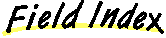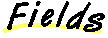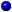`All Packages  Class Hierarchy  This Package  Previous  Next  Index`

# Class java.lang.Float

```java.lang.Object
|
+----java.lang.Number
|
+----java.lang.Float
```

public final class Float
extends Number
The Float class wraps a value of primitive type `float` in an object. An object of type `Float` contains a single field whose type is `float`.

In addition, this class provides several methods for converting a `float` to a `String` and a `String` to a `float`, as well as other constants and methods useful when dealing with a `float`.

##MAX_VALUE
The largest positive value of type `float`.MIN_VALUE
The smallest positive value of type `float`.NaN
The NaN value of type `float`.NEGATIVE_INFINITY
The negative infinity of type `float`.POSITIVE_INFINITY
The positive infinity of type `float`.TYPE
The Class object representing the primitive type float.

##Float(double)
Constructs a newly allocated `Float`object that represents the argument converted to type `float`.Float(float)
Constructs a newly allocated `Float` object that represents the primitive `float` argument.Float(String)
Constructs a newly allocated `Float` object that represents the floating- point value of type `float` represented by the string.

##byteValue()
Returns the value of this Float as a byte (by casting to a byte).doubleValue()
Returns the double value of this Float.equals(Object)
Compares this object against some other object.floatToIntBits(float)
Returns the bit represention of a single-float value.floatValue()
Returns the float value of this Float object.hashCode()
Returns a hashcode for this Float.intBitsToFloat(int)
Returns the single-float corresponding to a given bit represention.intValue()
Returns the integer value of this Float (by casting to an int).isInfinite()
Returns true if this Float value is infinitely large in magnitude.isInfinite(float)
Returns true if the specified number is infinitely large in magnitude.isNaN()
Returns true if this Float value is Not-a-Number (NaN).isNaN(float)
Returns true if the specified number is the special Not-a-Number (NaN) value.longValue()
Returns the long value of this Float (by casting to a long).shortValue()
Returns the value of this Float as a short (by casting to a short).toString()
Returns a String representation of this Float object.toString(float)
Returns a String representation for the specified float value.valueOf(String)
Returns the floating point value represented by the specified String.

##POSITIVE_INFINITY
``` public static final float POSITIVE_INFINITY
```
The positive infinity of type `float`.NEGATIVE_INFINITY
``` public static final float NEGATIVE_INFINITY
```
The negative infinity of type `float`.NaN
``` public static final float NaN
```
The NaN value of type `float`.MAX_VALUE
``` public static final float MAX_VALUE
```
The largest positive value of type `float`.MIN_VALUE
``` public static final float MIN_VALUE
```
The smallest positive value of type `float`.TYPE
``` public static final Class TYPE
```
The Class object representing the primitive type float.

##Float
``` public Float(float value)
```
Constructs a newly allocated `Float` object that represents the primitive `float` argument.

Parameters:
value - the value to be represented by the `Float`.Float
``` public Float(double value)
```
Constructs a newly allocated `Float`object that represents the argument converted to type `float`.

Parameters:
value - the value to be represented by the `Float`.Float
``` public Float(String s) throws NumberFormatException
```
Constructs a newly allocated `Float` object that represents the floating- point value of type `float` represented by the string. The string is converted to a `float` value as if by the `valueOf` method.

Parameters:
s - a string to be converted to a `Float`.
Throws: NumberFormatException
if the string does not contain a parsable number.
valueOf

##toString
``` public static String toString(float f)
```
Returns a String representation for the specified float value.

The values `NaN`, `NEGATIVE_INFINITY`, `POSITIVE_INFINITY`, `-0.0`, and `+0.0` are represented by the strings `"NaN"`, `"-Infinity"`, `"Infinity"`,` "-0.0"`, and `"0.0"`, respectively.

If `d` is in the range ```10-3 <= |d| <= 107```, then it is converted to a `String` in the style `[-]ddd.ddd`. Otherwise, it is converted to a string in the style `[-]m.ddddE±xx`.

There is always a minimum of 1 digit after the decimal point. The number of digits is the minimum needed to uniquely distinguish the argument value from adjacent values of type `float`.

Parameters:
d - the float to be converted.
Returns:
a string representation of the argument.valueOf
``` public static Float valueOf(String s) throws NumberFormatException
```
Returns the floating point value represented by the specified String.

Parameters:
s - the string to be parsed.
Returns:
a newly constructed `Float` initialized to the value represented by the `String` argument.
Throws: NumberFormatException
if the string does not contain a parsable number.isNaN
``` public static boolean isNaN(float v)
```
Returns true if the specified number is the special Not-a-Number (NaN) value.

Parameters:
v - the value to be tested.
Returns:
`true` if the argument is NaN; `false` otherwise.isInfinite
``` public static boolean isInfinite(float v)
```
Returns true if the specified number is infinitely large in magnitude.

Parameters:
v - the value to be tested.
Returns:
`true` if the argument is positive infinity or negative infinity; `false` otherwise.isNaN
``` public boolean isNaN()
```
Returns true if this Float value is Not-a-Number (NaN).

Returns:
`true` if the value represented by this object is NaN; `false` otherwise.isInfinite
``` public boolean isInfinite()
```
Returns true if this Float value is infinitely large in magnitude.

Returns:
`true` if the value represented by this object is positive infinity or negative infinity; `false` otherwise.toString
``` public String toString()
```
Returns a String representation of this Float object. The primitive `float` value represented by this object is converted to a `String` exactly as if by the method `toString` of one argument.

Returns:
a `String` representation of this object.
Overrides:
toString in class Object
toStringbyteValue
``` public byte byteValue()
```
Returns the value of this Float as a byte (by casting to a byte).

Overrides:
byteValue in class NumbershortValue
``` public short shortValue()
```
Returns the value of this Float as a short (by casting to a short).

Overrides:
shortValue in class NumberintValue
``` public int intValue()
```
Returns the integer value of this Float (by casting to an int).

Returns:
the `float` value represented by this object converted to type `int` and the result of the conversion is returned.
Overrides:
intValue in class NumberlongValue
``` public long longValue()
```
Returns the long value of this Float (by casting to a long).

Returns:
the `float` value represented by this object is converted to type `long` and the result of the conversion is returned.
Overrides:
longValue in class NumberfloatValue
``` public float floatValue()
```
Returns the float value of this Float object.

Returns:
the `float` value represented by this object.
Overrides:
floatValue in class NumberdoubleValue
``` public double doubleValue()
```
Returns the double value of this Float.

Overrides:
doubleValue in class NumberhashCode
``` public int hashCode()
```
Returns a hashcode for this Float.

Returns:
a hash code value for this object.
Overrides:
hashCode in class Objectequals
``` public boolean equals(Object obj)
```
Compares this object against some other object. The result is `true` if and only if the argument is not `null` and is a `Float` object that represents a `float` that has the identical bit pattern to the bit pattern of the `float` represented by this object.

Note that in most cases, for two instances of class `Float`, `f1` and `f2`, the value of `f1.equals(f2)` is `true` if and only if

``` f1.floatValue() == f2.floatValue() ```

also has the value `true`. However, there are two exceptions:

• If `f1` and `f2` both represent `Float.NaN`, then the `equals` method returns `true`, even though `Float.NaN==Float.NaN` has the value `false`.
• If `f1` represents `+0.0f` while `f2` represents `-0.0f`, or vice versa, the `equal` test has the value `false`, even though `0.0f==-0.0f` has the value `true`.

Returns:
`true` if the objects are the same; `false` otherwise.
Overrides:
equals in class Object
floatToIntBitsfloatToIntBits
``` public static native int floatToIntBits(float value)
```
Returns the bit represention of a single-float value. The result is a representation of the floating-point argument according to the IEEE 754 floating-point "single precision" bit layout.

Bit 31 represents the sign of the floating-point number. Bits 30-23 represent the exponent. Bits 22-0 represent the significand (sometimes called the mantissa) of the floating-point number.

If the argument is positive infinity, the result is `0x7f800000`.

If the argument is negative infinity, the result is `0xff800000`.

If the argument is NaN, the result is `0x7fc00000`.

Parameters:
value - a floating-point number.
Returns:
the bits that represent the floating-point number.intBitsToFloat
``` public static native float intBitsToFloat(int bits)
```
Returns the single-float corresponding to a given bit represention. The argument is considered to be a representation of a floating-point value according to the IEEE 754 floating-point "single precision" bit layout.

If the argument is `0x7f800000`, the result is positive infinity.

If the argument is `0xff800000`, the result is negative infinity.

If the argument is any value in the range `0x7f800001` through `0x7f8fffff` or in the range `0xff800001` through `0xff8fffff`, the result is NaN. All IEEE 754 NaN values are, in effect, lumped together by the Java language into a single value.

Parameters:
bits - an integer.
Returns:
the single-format floating-point value with the same bit pattern.

`All Packages  Class Hierarchy  This Package  Previous  Next  Index`

Submit a bug or feature